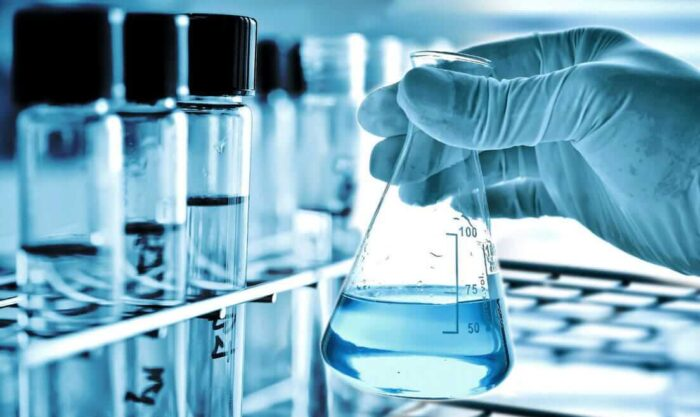The gaseous state of matter occurs between the liquid and plasma states, the latter of which provides the upper temperature boundary for gases. Bounding the lower end of the temperature scale lie degenerative quantum gases which are gaining increasing attention. High-density atomic gases super-cooled to very low temperatures are classified by their statistical behavior as either Bose gases or Fermi gases.

## Course Contents

• Ideal gas behaviour and deviations from it
• pV = nRT and its use in determining a value for Mr
• Dalton’s Law and its use in determining the partial pressures of gases in a mixture

## Learning Outcomes

Candidates should be able to:

• (a) state the basic assumptions of the kinetic theory as applied to an ideal gas
• (b) explain qualitatively in terms of intermolecular forces and molecular size:
• (i) the conditions necessary for a gas to approach ideal behaviour
• (ii) the limitations of ideality at very high pressures and very low temperatures
• (c) state and use the general gas equation pV = nRT in calculations, including the determination of Mr
• (d) use Dalton’s Law to determine the partial pressures of gases in a mixture (see also Section 9)